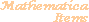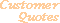Home Older Mathematica Items

Scientific Arts

Maxwellian Probability Distribution Function

Page 1 | Page 2 | Page 3 | Page 4

Inverse Series Method with Padé Approximants

We can attempt to fix the problem encountered above by extending the reach of the inverse series solutions. Often, when a truncated power series does not perform well beyond a particular point (perhaps because the function that the series approximates has a singularity that limits the radius of convergence) the technique of Padé approximants can make use of the data contained in the series coefficients in a more robust fashion. Padé approximants are rational function approximations to a given function. Mathematica contains a function that creates Padé approximants in the standard add-on package Calculus`Pade`. First load the package.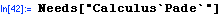Here is the usage message for Pade: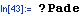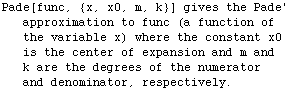So we define a function similar to InverseSeriesMaxwellCDF which makes use of Padé approximants. Here we make one of many possible choices by setting the degree of the numerator polynomial equal to that of the denominator.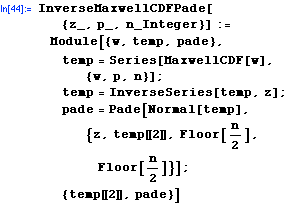There is one subtle point that involves the fact that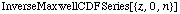gives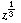times a series in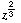.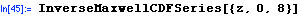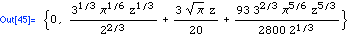The Padé algorithm needs a series in integer powers of the variable.  While we could factor out theand make a variable substitution prior to creating the Padé approximant, it is easier to just use the series expression for this special point.

Let's build the function. First make the Padé approximants to the inverse series.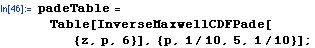Now append the expression for the series around 0.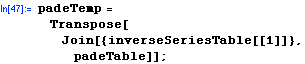Here is the function.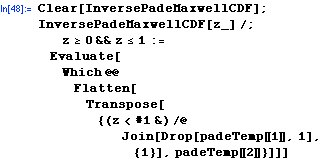Let's see how it compares with the InterpolationFunction approach.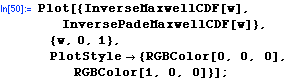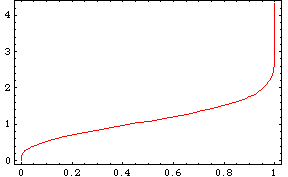The numerical problems near zero seem to have been resolved.

Here is a plot of the difference between the Padé method and the InterpolatingFunction method.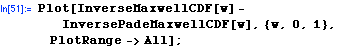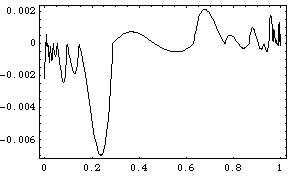Here is a timing test. The result is comparable to that for InverseSeriesMaxwellCDF above.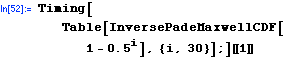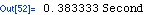<<Previous

 For further information on our services send email to info@scientificarts.com . Contents of this web site Copyright © 1999-2011, Scientific Arts, LLC.
 s